# Answers Practice Questions The Solid StateAnswers Practice Questions The Solid State

Ans 1. AgBr has both schottky and frenkel defects.  In general, silver halides show both Schottky and Frenkel defects. It is because AgBr crystal has a coordination number of 6 which is neither high nor low. The radius ratio of AgBr is also intermediate, thus AgBr satisfies the conditions to exhibit both types of defects.

Ans 2. (a). Packing Efficiency of Simple Cubic Unit Cell: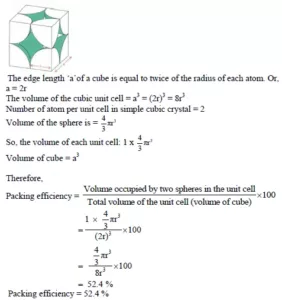(b). Packing Efficiency of Body Centred Unit Cell: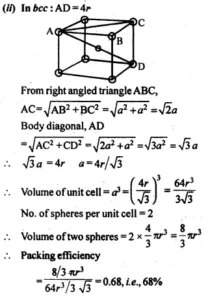(c). Packing Efficiency in Face Centred Unit cell: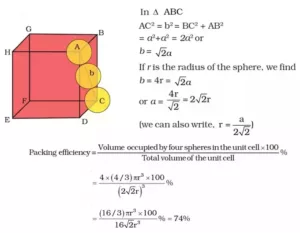Ans 3. The smallest repeating unit of the space lattice is Unit Cell.

Ans 4. Since p occupy tetrahedral void,
P= 2N and Q = N
2N P: N Q
2P:Q
The Formula of the Compound: P2Q1

Answers Practice Questions The Solid State

Ans 5.  Difference between Crystalline and Amorphous solids

 Crystalline solids Amorphous solids They have definite characteristic geometrical shape. They have an irregular shape. They have long-range order of regular pattern of arrangement of constituent particles. They have short-range order of regular pattern of arrangement of constituent particles. They are true solids. They are pseudo solids or supercooled liquids. They are anisotropic in nature. They are isotropic in nature.

Ans 6. Zinc oxide is white in colour at room temperature. On heating, it loses oxygen and turns yellow.
ZnO → Zn2+ + 1/2O2 + 2e
Now there is excess zinc in the crystal and its formula becomes Zn1+xO. The excess Zn2+ ions move to interstitial sites and the electrons to neighbouring interstitial sites.

Ans 7. In body-centered cube, 4r = 3​a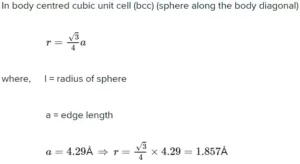Ans 8. It is given that the structure of mixed oxide is cubic closed packed.
Therefore, the number of O2− ions according to ccp = 4
So, the number of tetrahedral voids = 8 and octahedral voids = 4
Ions occupy 1/4​th of tetrahedral voids =2
B ions occupy all octahedral voids =4
A:B:O =2:4:4 =1:2:2
Hence, the formula is AB2​O2​.

Answers Practice Questions The Solid State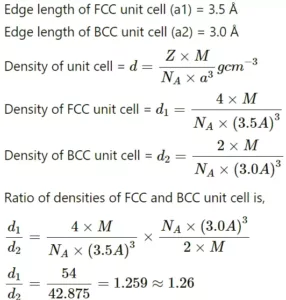Ans 11. (a).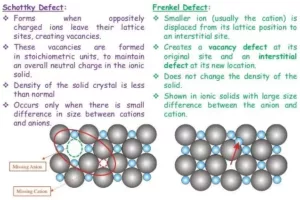(b). When a crystal of LiCl is heated in an atmosphere of Li vapour, the Li atoms lose an electron to form Li-ions. The released electrons diffuse into the crystal and occupy anionic sites ( F-centres ). These electrons impart a pink colour to the LiCl crystal.

(c). This defect usually occurs in ionic solids with a large size difference between the anion and cation.
LiCl does not exhibit Frenkel defect because the size difference between the anion and the cation of the compound is very small.

Answers Practice Questions The Solid State

Ans 12. (a). On the basis of their conductivities solids can be classified as conductors, insulators, and semiconductors. The difference between the three can be explained on the basis of band theory
Conductors: Solids having a magnitude of the range of conductivities from 104 to 107 ohm-1 m-1 are classified as conductors. Metals are good conductor of electricity. Silver has conductivity in the order of 107 ohm-1 m-1 is considered a very good conductor. In term of band theory, both valance band and conduction band gets overlap so electrons can easily migrate.
Semi-Conductors: Solids having an intermediate range of conductivity, i.e. from 10-6 to 104 ohm-1 m-1 are called semiconductors. In term of band theory, both valance band and conduction band are a little away from each other. The gap between the two is called the forbidden zone or bandgap overlap so electrons can easily migrate.
Insulators: Solids having a range of conductivity from 10-20 to 10-10 ohm-1 m-1 are considered as insulators. In term of band theory, there is a large gap between valance band and conduction band. So electrons cannot jump from the valence band to the conduction band.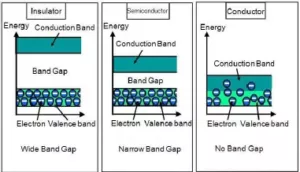(b). The charge on Cu will +1 in Cu2O and in a normal compound Cu has + 2 charge and every 2 ions of Cu+1 will be replaced by one ion of Cu+2. The conductance is arising due to these positive holes hence it will form a
p-type of semiconductor.

Answers Practice Questions The Solid State

Ans 13.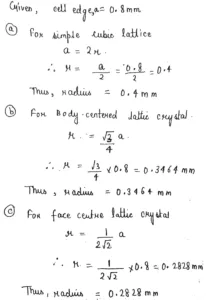Ans 14. We all know that oxygen like bigger molecules occupies the lattice points. The effective number of atoms in a CCP unit cell is 8 x 1/8 + 6 x 1/2 = 4
The total number of tetrahedral voids (X is present) in CCP is 8
Therefore no. of divalent ions = 8/8 = 1
Number of Octahedral voids (Y is present) = 4
1/2 of no. of divalent ion will be 4/2 = 2
Number of oxide = present at corners + at face centre = 8 x 1/8 + 6 x 1/2 = 4
So the formula of the compound will be AB2O4

Ans 15. The ratio of the ionic radius of the cation to the ionic radius of the anion is known as the radius ratio.
The radius ratio rule gives a relationship between the radius, coordination number and the structural arrangement of molecules in an atom.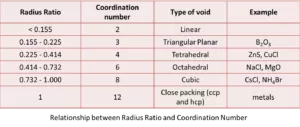Answers Practice Questions The Solid State